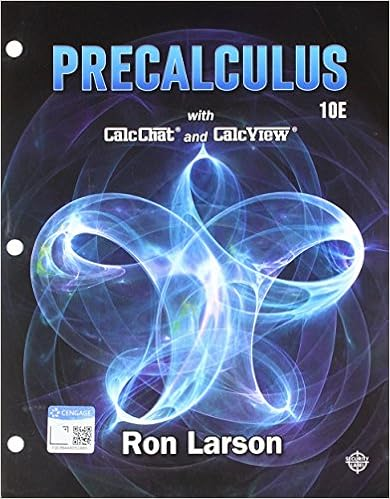# Exam 3 Practice Test - Exam Name MULTIPLE CHOICE Choose the...

• Test Prep
• RBSD44
• 10
• 89% (137) 122 out of 137 people found this document helpful

This preview shows page 1 - 3 out of 10 pages.

##### We have textbook solutions for you!
The document you are viewing contains questions related to this textbook.The document you are viewing contains questions related to this textbook.
Chapter 9 / Exercise 65
Precalculus
Hostetler/LarsonExpert Verified
ExamName___________________________________MULTIPLE CHOICE. Choose the one alternative that best completes the statement or answers the question.1)A ________ represents the width of the confidence interval between a sample mean and its upperlimit or between a sample mean and its lower limit.1)A)confidence intervalB)significance levelC)margin of errorD)confidence level
TRUE/FALSE. Write 'T' if the statement is true and 'F' if the statement is false.2)The alternative hypothesis, denoted by H1, represents the opposite of the null hypothesis and isbelieved to be true if the null hypothesis is found to be false.2)3)The sum of squares between (SSB) measures the variation between each sample mean and thegrand mean of the data.3)MULTIPLE CHOICE. Choose the one alternative that best completes the statement or answers the question.4)Consider the partially completed one-way ANOVA summary table.SourceSum ofSquares Degrees ofFreedomMean Sumof SquaresFBetween 270Within18Total81021The degrees of freedom for the sum of squares between for this ANOVA procedure is4)
5)The ________ provides an estimate for the population variance if the null hypothesis is true forone-way ANOVA.5)
Write 'T' if the statement is true and 'F' if the statement is false.6)The sum of squares within (SSW) measures the variation between each data value of the grandmean for all of the data.6)7)The degrees of freedom for the total sum of squares for one-way ANOVA equal the total number ofobservations minus two.7)1
##### We have textbook solutions for you!
The document you are viewing contains questions related to this textbook.The document you are viewing contains questions related to this textbook.
Chapter 9 / Exercise 65
Precalculus
Hostetler/LarsonExpert Verified
MULTIPLE CHOICE. Choose the one alternative that best completes the statement or answers the question.8)The ________ provides an estimate for the population variance whether or not the null hypothesisis true for one-way ANOVA.8)
9)The sum of squares ________ measures the variation between each sample mean and the grandmean of the data.9)A)blockB)withinC)betweenD)error
10)Two real estate companies, Century 21 and RE/MAX, compete with one another in a local market.The manager of the Century 21 office would like to advertise that homes listed with RE/MAXaverage more than 10 days on the market when compared to homes listed with his company. Thefollowing data shows the sample size and average number of days on the market for the twocompanies along with the population standard deviations.Century 21RE/MAXSample mean122 days144 daysSample size3630Population standard deviation32 days35 daysIf Population 1 is defined as RE/MAX and Population 2 is defined as Century 21, and using ΅0.10, the conclusion for this hypothesis test would be because the test statistic is10)=
•••